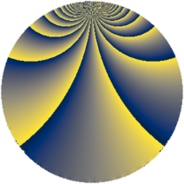# Properties

 Label 900.3.mLevel $900$ Weight $3$ Character orbit 900.m Rep. character $\chi_{900}(107,\cdot)$ Character field $\Q(\zeta_{4})$ Dimension $144$ Sturm bound $540$

# Related objects

## Defining parameters

 Level: $$N$$ $$=$$ $$900 = 2^{2} \cdot 3^{2} \cdot 5^{2}$$ Weight: $$k$$ $$=$$ $$3$$ Character orbit: $$[\chi]$$ $$=$$ 900.m (of order $$4$$ and degree $$2$$) Character conductor: $$\operatorname{cond}(\chi)$$ $$=$$ $$60$$ Character field: $$\Q(i)$$ Sturm bound: $$540$$

## Dimensions

The following table gives the dimensions of various subspaces of $$M_{3}(900, [\chi])$$.

Total New Old
Modular forms 768 144 624
Cusp forms 672 144 528
Eisenstein series 96 0 96

## Trace form

 $$144q + O(q^{10})$$ $$144q - 112q^{16} - 112q^{22} - 32q^{28} - 16q^{37} + 320q^{46} + 488q^{52} + 400q^{58} + 256q^{61} + 224q^{73} - 1152q^{76} - 960q^{82} - 544q^{88} + 160q^{97} + O(q^{100})$$

## Decomposition of $$S_{3}^{\mathrm{new}}(900, [\chi])$$ into newform subspaces

The newforms in this space have not yet been added to the LMFDB.

## Decomposition of $$S_{3}^{\mathrm{old}}(900, [\chi])$$ into lower level spaces

$$S_{3}^{\mathrm{old}}(900, [\chi]) \cong$$ $$S_{3}^{\mathrm{new}}(60, [\chi])$$$$^{\oplus 4}$$$$\oplus$$$$S_{3}^{\mathrm{new}}(180, [\chi])$$$$^{\oplus 2}$$$$\oplus$$$$S_{3}^{\mathrm{new}}(300, [\chi])$$$$^{\oplus 2}$$